In order to calculate the angular distribution of the energy radiated by an accelerated charge, we must think carefully about what is meant by the rate of radiation of the charge. This quantity is actually the amount of energy lost by the charge in a retarded time interval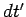during the emission of the signal. Thus,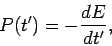(1657)

whereis the energy of the charge. The Poynting vector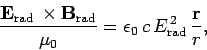(1658)

where use has been made of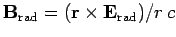[see Eq. (1619)], represents the energy flux per unit actual time,. Thus, the energy loss rate of the charge into a given element of solid angle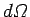is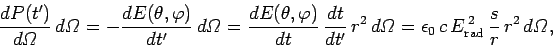(1659)

where use has been made of Eq. (1605). Here,and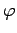are angular coordinates used to locate the element of solid angle. It follows from Eq. (1625) that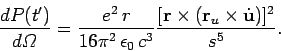(1660)

Consider the special case in which the direction of acceleration coincides with the direction of motion. Let us define spherical polar coordinates whose axis points along this common direction. It is easily demonstrated that, in this case, the above expression reduces to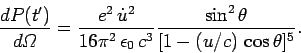(1661)

In the non-relativistic limit,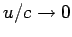, the radiation pattern has the familiardependence of dipole radiation. In particular, the pattern is symmetric in the forward (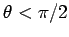) and backward (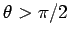) directions. However, as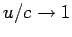, the radiation pattern becomes more and more concentrated in the forward direction. The angle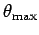for which the intensity is a maximum is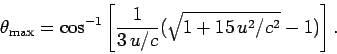(1662)

This expression yields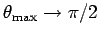as, and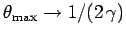as. Thus, for a highly relativistic charge, the radiation is emitted in a narrow cone whose axis is aligned along the direction of motion. In this case, the angular distribution (1661) reduces to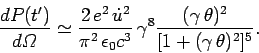(1663)

The total power radiated by the charge is obtained by integrating Eq. (1661) over all solid angles. We obtain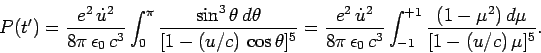(1664)

It is easily verified that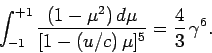(1665)

Hence,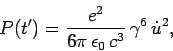(1666)

which agrees with Eq. (1649), provided that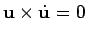.